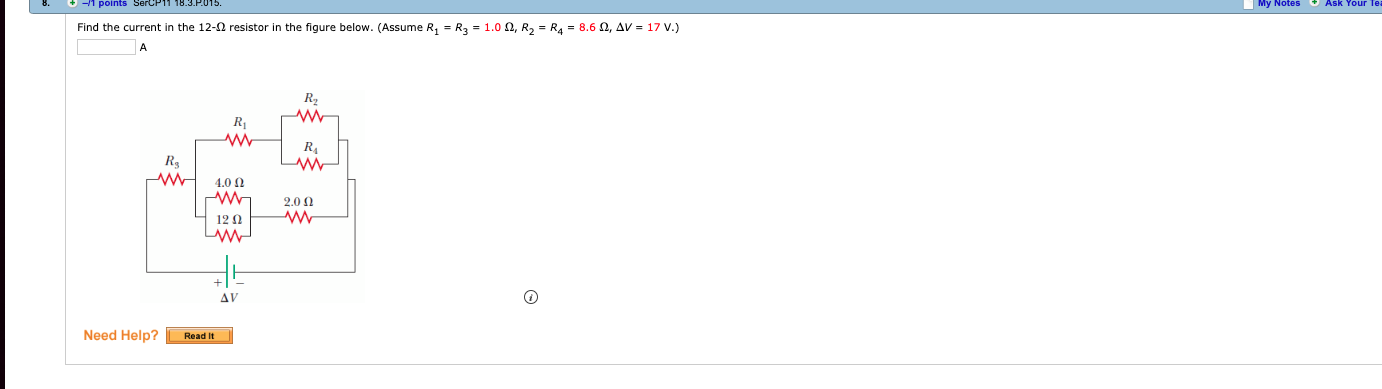# 8.-1 points SercP11 18.3 P.015My Notes Ask Your Te|Find the current in the 12-Ω resistor in the figure below. (Assume R1-R3 = 1.0 Ω, R2-R4-8.6 Ω, Δν = 17 V.)R2Rsw 40012ΩNeed Help? Resdit

Question
42 viewshelp_outlineImage Transcriptionclose8. -1 points SercP11 18.3 P.015 My Notes Ask Your Te | Find the current in the 12-Ω resistor in the figure below. (Assume R1-R3 = 1.0 Ω, R2-R4-8.6 Ω, Δν = 17 V.) R2 Rs w 400 12Ω Need Help? Resdit fullscreen
check_circle

Step 1

Resistances R4 and R2 are connected in parallel. Hence,

Step 2

Resistances R24 and R1 are in series. Hence,

Step 3

Parallel combination of 4.0 Ω and 12 &Omeg...

### Want to see the full answer?

See Solution

#### Want to see this answer and more?

Solutions are written by subject experts who are available 24/7. Questions are typically answered within 1 hour.*

See Solution
*Response times may vary by subject and question.
Tagged in

### Physics